## Introduction

My wife and I got married a little less than a year ago, and we’re starting to consider the life that we can build together. Like most couples, we’ve been trying to think through activities such as starting a family, buying a home, and possible career changes; and how to make sure that we can achieve these things without working too hard, so that we can have time to enjoy the fruits of our labour. Naturally, forward projection is an incredibly difficult task with many different variables, many of which are difficult to anticipate. My standard way of trying to make sense of this all involves trying to reason through mean values and making a wide range of assumptions, many of which have turned out to be pretty poor.

Ultimately, it clicked that I might want to consider many trajectories, each the result of a stochastic process, such that I could form a statistical distribution. With this modeling technique in hand, I could start to answer questions such as “What size house can we afford to reliably buy, if we’re targeting a due date of fall 2019”, with an answer like “99.7% of the time, our cash on hand is projected to be remain positive with a purchase price of $500,000” . The rest of this post is organized as follows: we start by discussing a simple stochastic process in python, and then we discuss building individual models for the major features of life that affect cash flow; before finally combining all of the models together and briefly discussing their interpretive power. ## Simulating Some Simple Stochastic Processes ### The Wiener Process The Wiener process, known also as Brownian motion, is a simple continuous time stochastic process that in applications as different as path integrals in quantum field theory, control theory, and finance. The theory behind the process was originally developed to model the motion of a particle in a fluid medium, where the particle undergoes random motion due to the random fluctuations of the medium. This motion was first observed by Robert Brown, when he observed the motion of a particle in water through a microscope and observed strange random motion. While originally developed to study the motion of a particle in a randomly fluctuating medium, models of Brownian motion have proven to be successful in finance, where the “medium” at hand is the market or other minor fluctuations that are otherwise impossible to capture. Given that household finances will have many of these same random fluctuations, this is a good starting point. In this discussion we will denote the Wiener process as$W$, which has individual elements$W_t$where the subscript$t$indicates the time index. This process has four simple properties: 1.$W_0 = 0$2.$W_{t + u} - W_t \sim \mathcal{N}(0, u) \sim \sqrt{u} \mathcal{N}(0, 1)$, where$\sim$indicates “drawn from”. 3. For$0 \le s<t<u<v \le T$,$W_t - W_s$and$W_v - W_u$are independent 4.$W_t$is continuous in$t$Using property two, we can immediately discretize this process by choosing to simulate: $W_{t + dt} - W_{t} \sim \sqrt{dt} \mathcal{N}(0, 1)$ which we will represent compactly as $dW_t \sim \sqrt{t} \mathcal{N}(0, 1).$ Then, to recover$W$, we simply use the cumulative sum: $W_t = \sum_{s \le t} dW_s.$ Computationally, this can be done easily using python and numpy in the following way: import numpy as np N = 100000 dt = 1. dW = np.random.normal(0., 1., int(N)) * np.sqrt(dt) W = np.cumsum(dW)  I’ve plotted five different Wiener process trajectories in Figure 1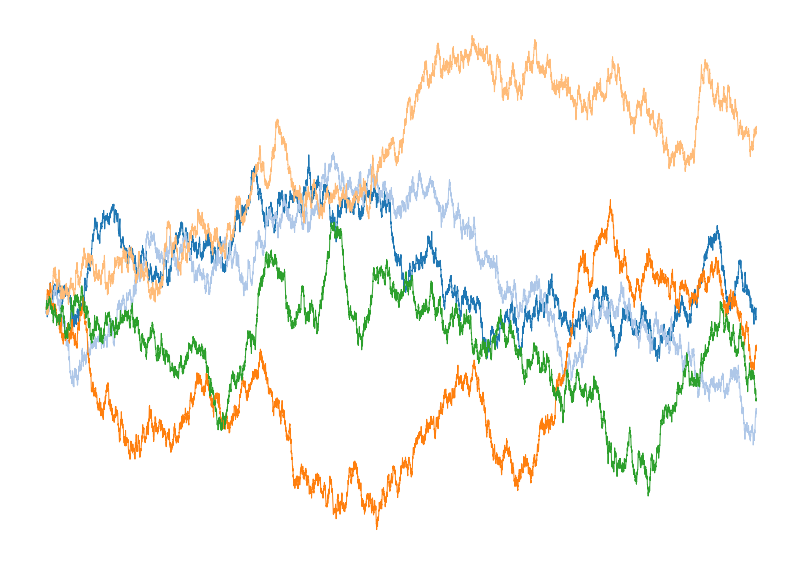Figure 1: Plot of five different instantiations of the discretized Wiener process generated using the code above. ### Geometric Brownian Motion With the Wiener process in hand, we can next explore a slightly more complicated stochastic processes named Geometric Brownian motion (GBM). Like a Wiener process, GBM is a continuous time stochastic process. GBM, however, assumes a logarithmic dependence on an underlying Wiener process with drift. The equation for such a process is $dS_t = \mu S_t dt + \sigma S_t dW_t,$ where$S_t$provides the time index for the value of the stochastic process,$\mu$is the constant related to the drift,$\sigma$represents the volatility of the process, and$W_t$is the Weiner process. This model is simple enough to be solved analytically, with the solution being: $S_t = S_0 e^{\left(\mu - \frac{\sigma^2}{2}\right) t + \sigma W_t}$ but this does not limit the applicability of GBM. In fact, GBM has seen applications in a wide range of fields including finance through the celebrated Black-Scholes model and other financial models, molecular dynamics, and even genetics. GBM is popular in all of these areas because it is intuitively easy to grasp, and easy to modify to include new physics such as stochastic jumps or non-constant volatility. While an analytic solution is useful, we will compute trajectories of the GBM by discritizing it on a grid, and using the cumulative summation that we used above for the Wiener process. In code, this looks like: import numpy as np def GBM(N=10000, mu=1.0, sigma=0.8, s0 = 1.0): t = np.linspace(0.,1.,N+1) dt = 1./N dW = np.random.normal(0., 1., int(N + 1)) * np.sqrt(dt) W = np.cumsum(dW) term1 = (mu - sigma**2/2.0) * t term2 = sigma * W S = s0 * np.exp(term1 + term2) return t, W, S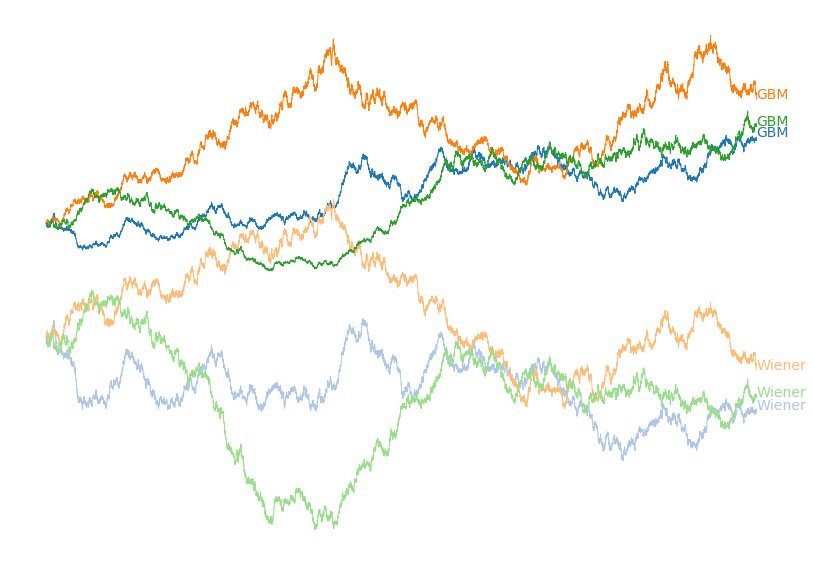Figure 2: Plot of three different instantiations of the discretized Geometric Brownian motion process and the underlying Wiener process generated using the code above. Matching colours have the same underlying stochastic processes. To model real world data with a GBM we can compute the drift and volatility from the data at hand, and then project forward many trajectories to understand the likelihood of many future possibilities. ## Building a model of Cash on Hand With an understanding of some stochastic processes in hand, we next seek to actually model personal financial data. One of the most important values to track when evaluating financial health is cash on hand. Obviously you generally want to spend less than your income, but how much less? And what are the effects of activities like buying a house, increasing rent, raises, having kids, vacations, and other life events on cash on hand? The most straight forward starting point is to model personal spending habits. Having analyzed my last 6 years of spending, they been constant with minor fluctuations once major life events such as paying for a wedding were subtracted. There also rarely appears to be any obvious memory from month to month. Because I mostly observed fluctuation of a calculable standard deviation around a calculable mean, with no historical dependence, we seem to meet most of the conditions for Wiener process model to hold. To build out this model, let’s assume an after-tax salary of$1000/month and a year of spending data that looks like the following:

[ $1106.95$819.1    $782.45$629.3    $951.48$795.21   $955.4$1004.88 $1109.47$1277.65  $1380.51$1058.21]


We can then compute the mean and standard deviations to arrive at data driven values for our financial model. This can be simply done by:

mean = np.mean(spending) # $989.22 std = np.std(spending) #$206.37


We can then generate a single forward trajectory of cash on hand with these values using the following code, which is closely related to the previous code:

N = 60 # 5 Years
dW = salary - np.random.normal(mean, std, int(N))
W = np.cumsum(dW)


where $W$ now represents our time dependent cash on hand. We can generate a single trajectory, but this is hardly going to be a valuable exercise because the odds of accurately predicting future cash on hand is extraordinarily low. Instead, we can understand the result of the trajectories in distribution by running many trajectories and exploring their distribution, which we have plotted below in Figure 4: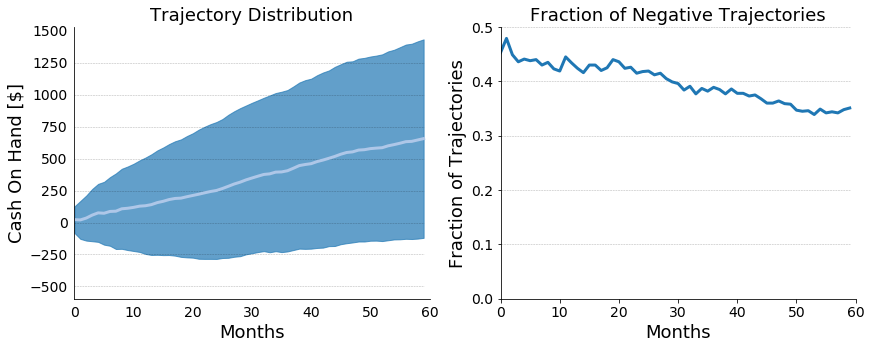Figure 3: On the left, a plot of projected cash on hand for our hypothetical financial scenario. The mean trajectory is plotted in white, and the standard deviation is plotted in blue. On the right, a plot of the fraction of trajectories with a negative cash on hand.

Month Mean [$] Notes Jan 2000 Feb 2000 Mar 2000 Apr 2000 May 2000 Jun 4000 Vacation Jul 2000 Aug 2500 Back to School Sep 2000 Oct 2000 Nov 3500 Christmas Plans Booked Dec 3000 Christmas Gifts With a simple modification, we can just plug these values right in: dW = [] salary = 3000 for i in range(N): monthly_spending = np.random.normal(SpendingMeans[i % 12], 300., 1) dW.append(salary - monthly_spending) W = np.cumsum(dW)  We can simulate just like before, and we can study distributions in the way to understand our HP2’s potential envelope of futures. These distributions are plotted in Figure 5: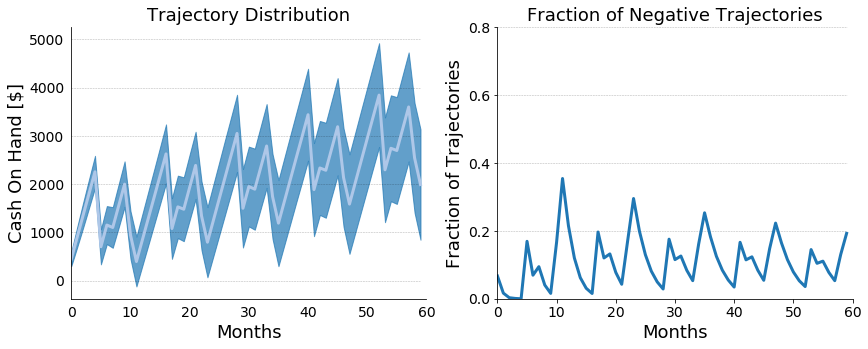Figure 4: On the left, a plot of projected cash on hand for our hypothetical financial scenario with time dependent means. The mean trajectory is plotted in white, and the standard deviation is plotted in blue. On the right, a plot of the fraction of trajectories with a negative cash on hand. ### Modeling Salaries With Stochastic Jump Process Up until now we’ve provided a somewhat coarse model for household spending but we’ve ignored the general stochasticity of compensation that comes from raises and bonuses. Over a multiyear projection, ignoring these compensation increases can lead under-forecasting of cash on hand. For the sake of modeling, I’m going to assume that our hypothetical person 2 (HP2) is a salaried individual, who is making 3000/month after taxes and averages a 4% raise year over year with a standard deviation of 2%. This volatility can come from market conditions, HP2’s individual performance, or performance of his employer. Whatever the case, capturing this variability will have a drastic impact on the accuracy of our cash on hand simulations. To do this we will start by assuming a yearly raise cycle, where the raise amount is drawn from a normal distribution that is constrained to be positive. mean_raise = 4.0 # Can be computed std_raise = 1.0 # Can be computed base_salary = 3000 # per year p = 1.0 # Base percentage salary = np.array([base_salary] * N) for i in range(len(salary)): if (i != 0) and (i % 12) == 0: p = p * (1. + np.abs(np.random.normal(mean_raise, std_raise))/100) salary[i] = salary[i] * p  Year Mean Yearly Salary 0 29400.0$\pm$0.0 1 30577.0$\pm$293.61 2 31802.77$\pm$431.88 3 33075.85$\pm$551.76 4 34397.68$\pm$662.16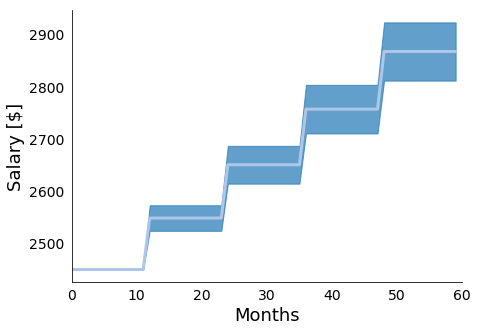Figure 5: In Figure 5 we present the mean and standard deviations of 10000 simulations of the presented yearly raise model. We see the expected stair step pattern, with more uncertainty as we project further into the future. With this simple model in hand, we will next assume stochasticity with respect the interval between raises. This distribution matches roughly the raise and promotion cycle that we generally experience in our jobs. That is, generally our raises correspond to yearly performance reviews, but promotions and raise cycles can come a touch earlier or a touch later. We will build this stochasticity in by drawing the periods between raises from a Poisson distribution with rate$\lambda$and a linear shift. To provide an intuition for what the Poisson distribution looks like, I’ve plotted a histogram of 100000000 samples below in Figure 6. def poisson_draw(lam = 2, shift = 0, truncate = False): while(1): val = shift + np.random.poisson(lam) if not truncate or (truncate and val >= 0): break return val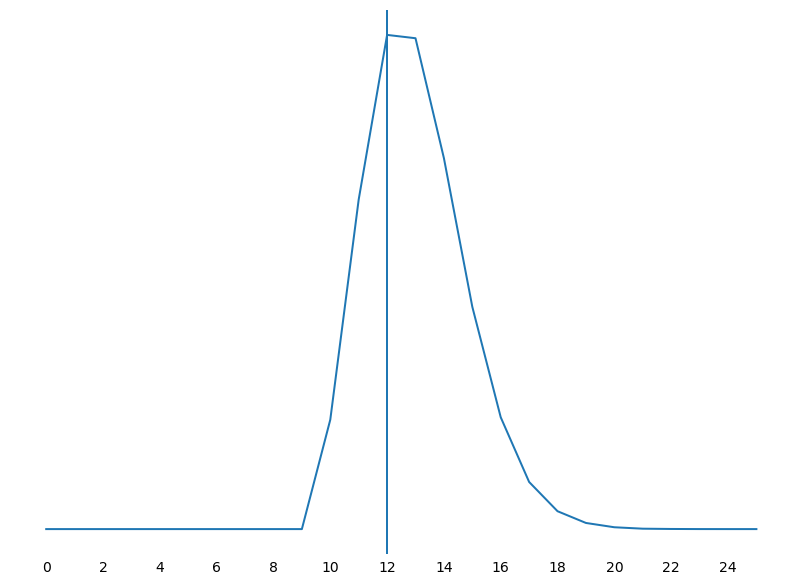Figure 6: In Figure 6 we present the poisson histogram that is generated by drawing 100000000 samples from the above function with lam = 3 and shift = 10. As expected, we see our most likely period as 12 months but we have some statistical support around a slightly early raise or a delayed raise, with the delay being more likely than the early raise. With a duration model in hand, we can then modify our simple stochastic raise model to include a stochastic time dependence between raises. This can be done simply by drawing the raise period from a poisson distribution, as indicated below:  last_raise = 0 raise_period = poisson_draw(lam = 4, shift = 8) for i in range(len(salary)): if (i != 0) and (i - last_raise) == raise_period: pp = np.abs(np.random.normal(mean_raise, std_raise))/100. p = p * (1. + pp) last_raise = i raise_period = poisson_draw(lam = 4, shift = 8) percentage.append(p) for i in range(len(salary)): salary[i] = salary[i] * percentage[i]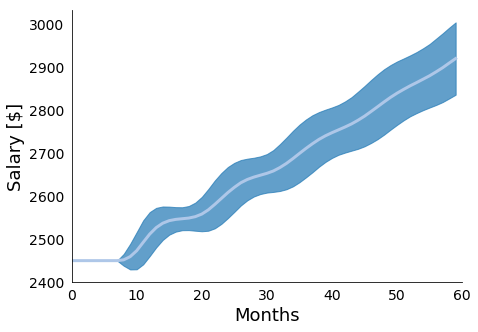Figure 7: In Figure 7 we present the mean and standard deviations of 10000 simulations of the presented raise model with stochastic raise periods. We see the stair step pattern gets "smeared" out due to the added uncertainty, with more uncertainty as we project further into the future. Year Mean Yearly Salary 0 29475.97$\pm$108.38 1 30614.07$\pm$369.22 2 31825.85$\pm$533.17 3 33093.98$\pm$671.24 4 34416.07$\pm\$ 803.32

The second major source of randomness in HP2’s compensation is the role of bonuses in total compensation. Bonuses typically fall within a range of percentage of salary, and are typically offered at the end of the year, which is how we will choose to model them in this case. Specifically, we will assume that HP2 has a 5% yearly bonus optionality, and that HP2 is bonused every December. This model is probably the simplest that we have to implement, and the code looks something like:

def bonus_model(bonus_mean, bonus_std, yearly_salary):
N = len(yearly_salary)
bonus_percentages = np.random.normal(bonus_mean, bonus_std, N)/100.
bonus = []
time = []
i = 0
for j in range(12*N):
time.append(j)
if (((j % 12) == 11) and j != 0):
bonus.append(bonus_percentages[i] * yearly_salary[i])
i = i + 1
else:
bonus.append(0.0)
time = np.array(time)
bonus = np.array(bonus)
return bonus, time


### Putting It All Together

Because our observable is cash, we can combine all of these models with through simple addition. This allows us to represent our total compensation model as:

salary, yearly_salary = stochastic_raise_salary_model(base_salary, mean_raise, std_raise, N)
bonus, months = bonus_model(mean_bonus, std_bonus, yearly_salary)
total_comp = (salary + bonus)


Putting this all together, we can combine the cyclic spending model with the above total compensation model to arrive at a richer set of simulations, which we present in figure 6.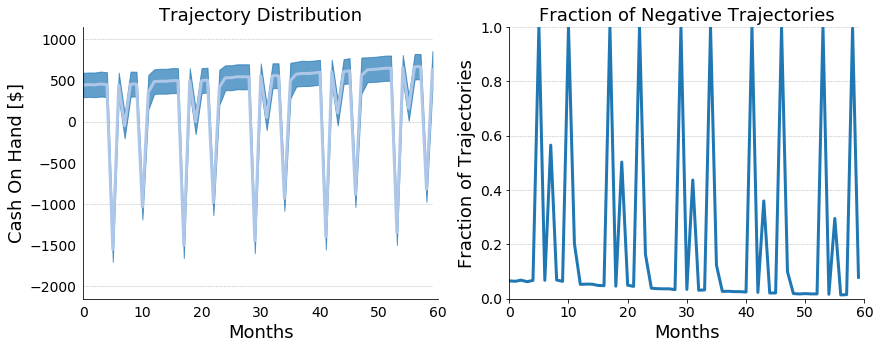Figure 8: In Figure 8 we present the cash on hand model that combines together the stochastic salary model with stochastic raise periods, stochastic bonus model, and cyclic stochastic spending model. Taken together we see that over the 5 year period our HP2 slowly becomes more financially stable, but still caries significant debt year after the holidays every year.

## Conclusions

These models were extremely simple but can be combined and extended with more parameters to account for deterministic things such as event based tax benefits (e.g. the interest deduction from buying a house), stock market performance, credit card debt, and other important events. I’ve done precisely this and they’ve already helped me to understand my finances more completely. Beyond this, they’ve also helped to reduce my overall anxiety around finances because uncertainty is a first class citizen of this approach.

Of course, beyond just extending these models, we also want to evaluate them and then use real-world data to inform them so that we can plan using a true posterior rather than just trying to draw inferences from our prior. I’ve chosen to do this personally by likelihood weighting my trajectories. This is commonly known as Approximate Bayesian Computation (ABC), and a followup post will detail how I used ABC by implementing a very special purpose probabilistic programming language.

 Please also note that any dollar figures quoted here are purely hypothetical due to the highly private nature of personal finances. This does not affect our modeling techniques, however.